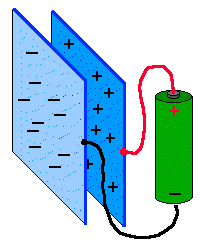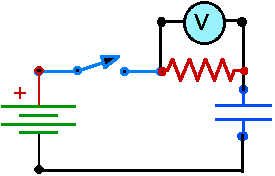# Charging a Capacitor.

Capacitors store electrical energy by separating charge. The LVPS is a source of voltage for moving charge, so it can be used to "charge " a capacitor. You know that there are two kinds of electrical charge, called negative and positive. Capacitors store equal quantities of both at the same time. The simplest capacitor is a pair of conducting plates separated with an insulator (dielectric). A voltage source can move negative charges (electrons) from one plate to another. The plate which looses electrons becomes positive and the plate with the extra electrons becomes negative. Current (Amperes) represents the flow of positive charge. As the electrons are pulled to the negative plate the current is represented as flowing to the positive plate. Of course electrons are repeled from the negative plate, so a voltage is needed to push them on forcibly. As more electrons are shoved onto the negative plate, that plate becomes more negative and it becomes more and more difficult to add electrons. This means that the current will slow down and eventually stop. When the electric field between the plates balances the voltage of the battery (or LVPS), no more current will flow.Figure E2
Moving Charges

The general question of this investigation is: "How long does it take to charge a capacitor?". Of course this is a question you can't answer because it is not specific enough. What specific questions can you ask?

Big currents will charge small capacitors too quickly to observe, so use resistors to slow the current flow. Inexpensive capacitors usually have very small values, in the microfarad range. To slow down charging so it can be investigated, resistors with big resistance values are necessary, The resistors for your circuit should be in the megohm range.

To determine the amount of charge flowing (current) from one capacitor plate to the other, you can measure the voltage drop across the resistor. I = V/RFigure E2
Charging a capacitor

Here are some things to try:

• Set up a charging circuit and determine how long it takes to charge some capacitor.
• Try this with a variety of capacitors and resistors
• The charging current is a transient, it changes and eventually fall to zero. Just how does this current change as time goes on? Measure it and graph it. If your capacitor charges too quickly then change the charging circuit. Does the charging voltage make a difference?
• Try combinations of capacitors. Connect two in series, try two connected in parallel. Does the charging time increase or decrease? Does the capacity of the combined capacitors get bigger or smaller?

Previous Page || Up a Level || Index || Next Page